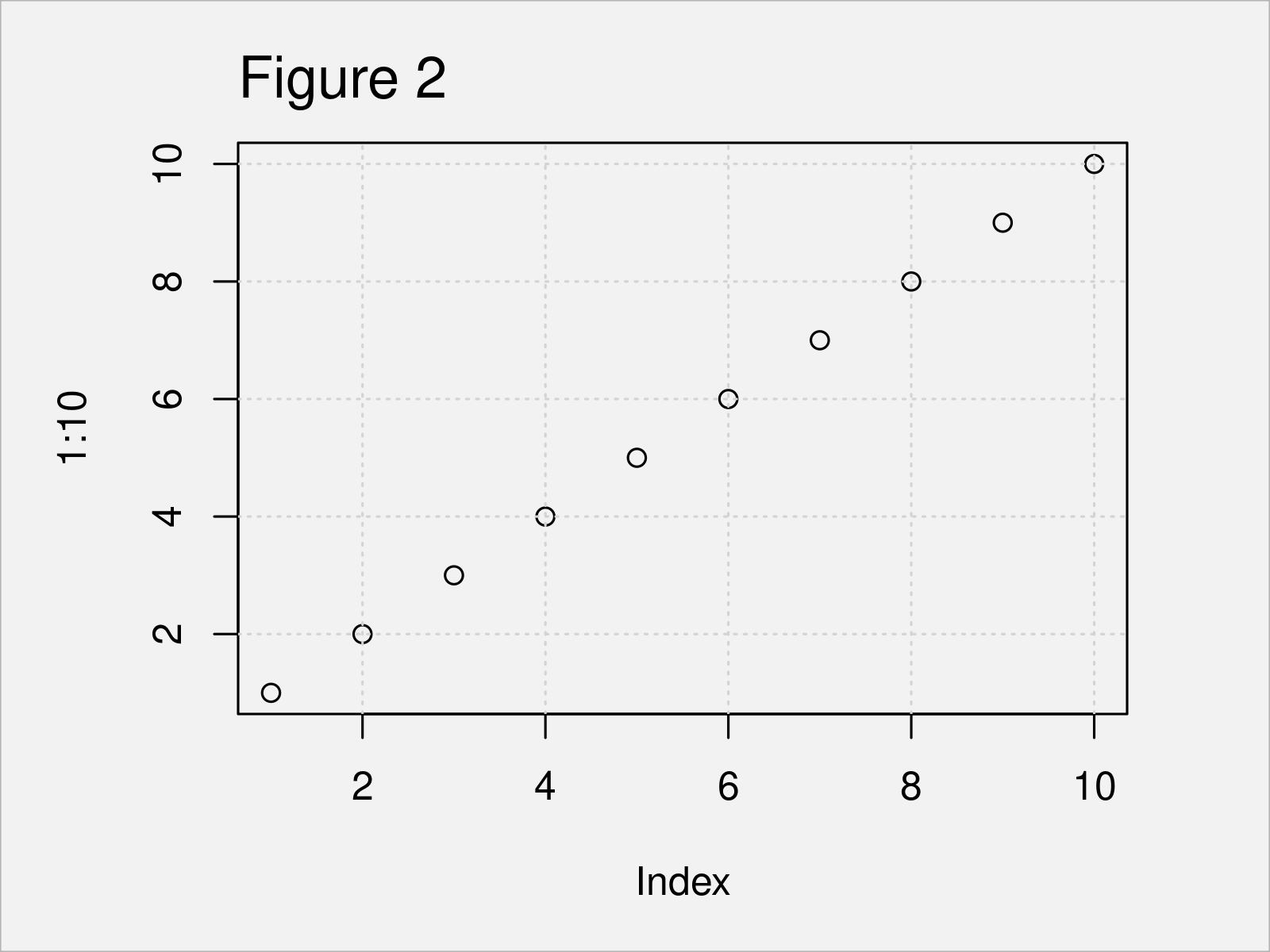# Add Grid Line Consistent with Ticks on Axis to Plot in R (2 Examples)

In this post, I’ll demonstrate how to draw a grid with tick marks on the corresponding default axis in R programming.

Let’s do this…

## Example 1: Add Grid with Certain Number of Lines to Plot

This example demonstrates how to draw a particular number of grid lines to a Base R plot.

For this, we can use the plot and grid functions as shown below:

```plot(1:10)                           # Draw plotIn Figure 1 it is shown that we have created a plot with grid lines by executing the previous R programming syntax.

However, you can also see that these grid lines are not aligned to the tick marks of our plot. In the next example, I’ll explain how to do that!

## Example 2: Align Grid with Tick Marks on Corresponding Default Axis

Example 2 demonstrates how to align grid lines to the tick marks of a Base R graph.

For this, we have to specify NULL within the grid function as shown below:

```plot(1:10)                           # Draw plotAfter running the previous syntax the plot with grid lines aligned to the axis tick marks shown in Figure 2 has been created.

## Video, Further Resources & Summary

If you need further explanations on the R programming code of this tutorial, I recommend having a look at the following video on my YouTube channel. I explain the R syntax of this article in the video.

Please accept YouTube cookies to play this video. By accepting you will be accessing content from YouTube, a service provided by an external third party.If you accept this notice, your choice will be saved and the page will refresh.

In addition, you could have a look at the other tutorials on this website.

At this point in the article you should have learned how to add a grid to a graphic with tick marks on the corresponding default axis in R. Please let me know in the comments section below, if you have any further questions.

Subscribe to the Statistics Globe Newsletter